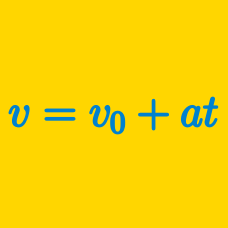Classical Mechanics

# Position-time graph - constant acceleration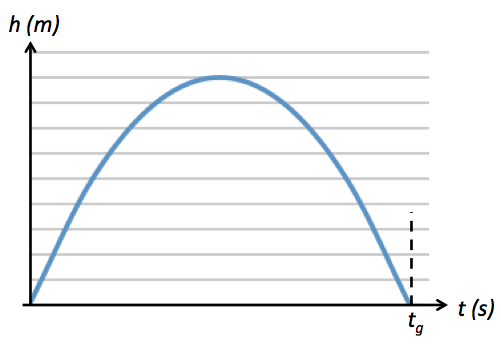The above graph depicts the height of a $200 \text{ g}$ ball thrown vertically upwards. If the ball falls back and hits the ground at $t_g=6 \text{ s},$ how fast was the ball thrown?

The gravitational acceleration is $g= 10 \text{ m/s}^2.$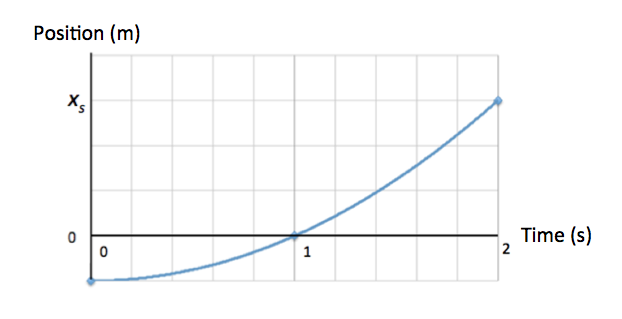The figure above depicts the motion of a particle moving along the $x$-axis with constant acceleration. The figure's vertical scaling is set by $x_s = 6 \text{ m}.$ Assuming that particle $A$ keeps moving in the same manner, what is the position of the particle at $t= 3 \text{ s}?$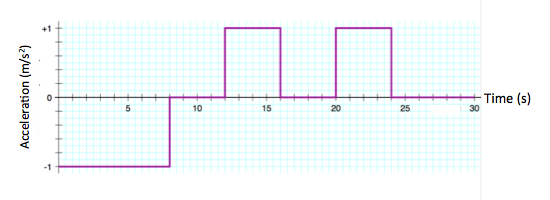The above graph depicts the acceleration of an object moving along the $x$-axis. If the object is initially at rest, what is the position of the object at $t = 30 \text{ s}?$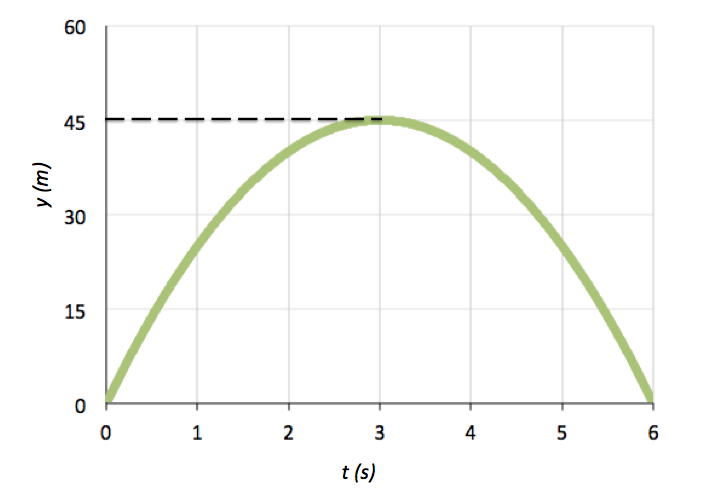A ball is shot vertically upward from the surface of a planet. A plot of $y$ versus $t$ for the ball is shown in the above graph, where $y$ is the height of the ball in meters above the ground and $t=0$ is the instant the ball is shot. What is the magnitude of free-fall acceleration of the planet?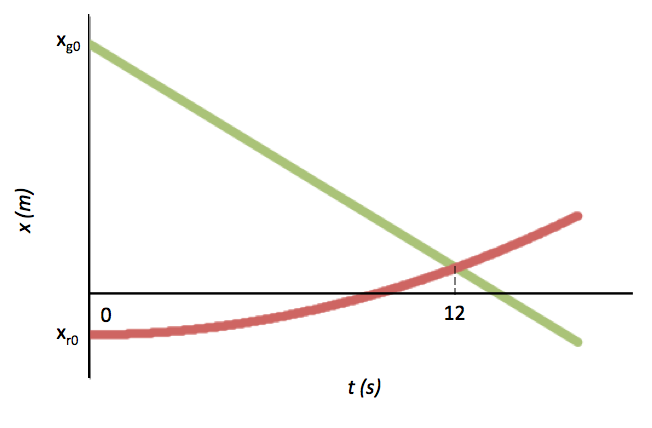A green car and a red car that are equal in mass are moving toward each other in adjacent straight lanes parallel to each other. As shown in the above graph, their respective initial positions at time $t= 0$ are $x_{g0} = 270 \text{ m}$ and $x_{r0} =-258\text{ m}.$ The green car has a constant speed of $20 \text{ m/s},$ while the red car begins from rest and runs in uniform acceleration motion. If the two cars pass each other at $t = 12.0 \text{ s},$ what is the magnitude of acceleration of the red car?

×